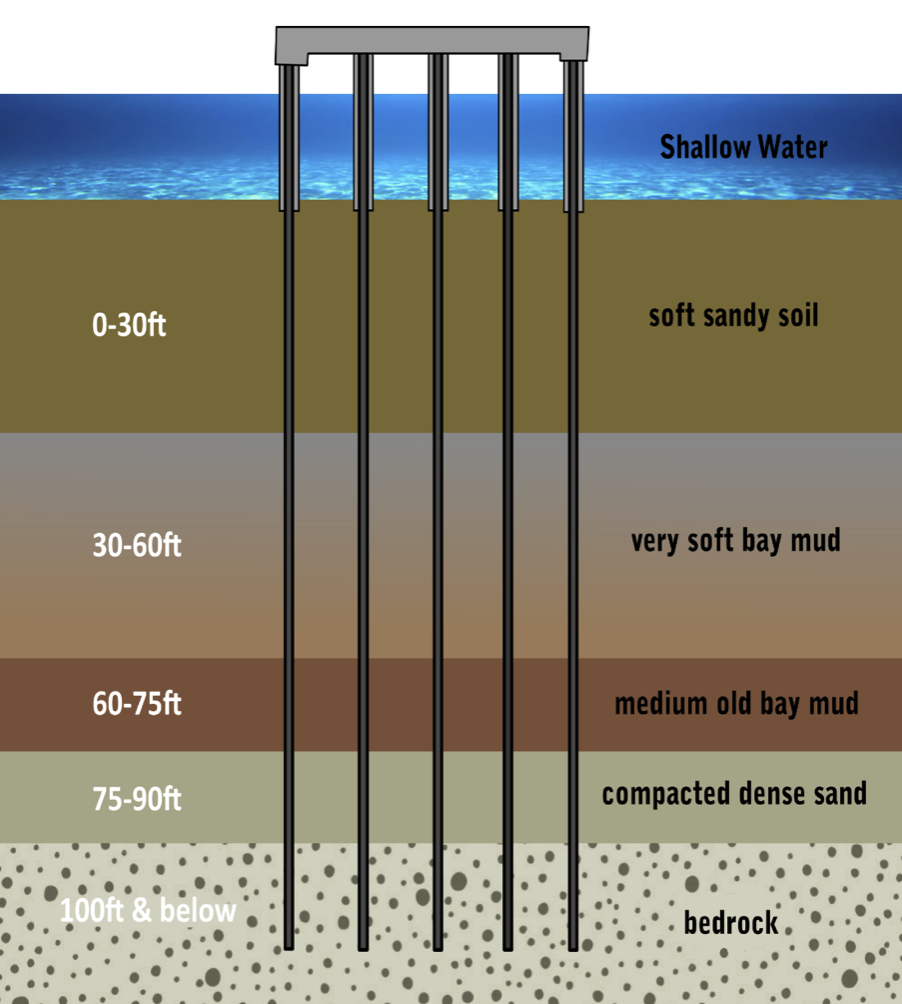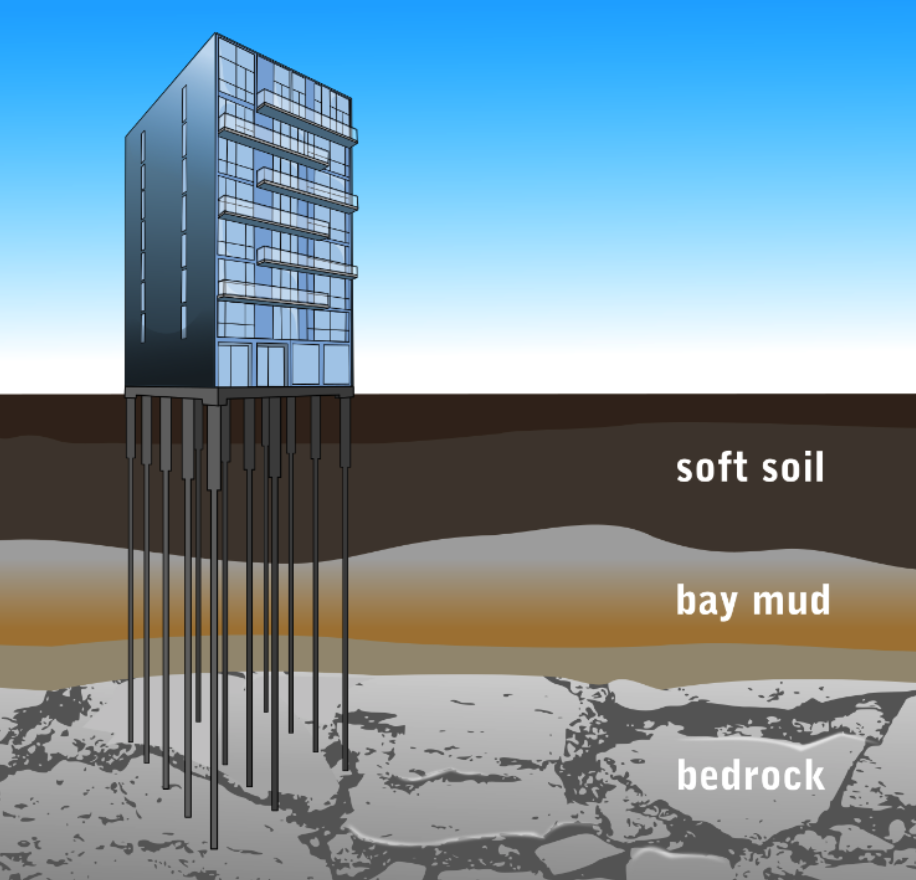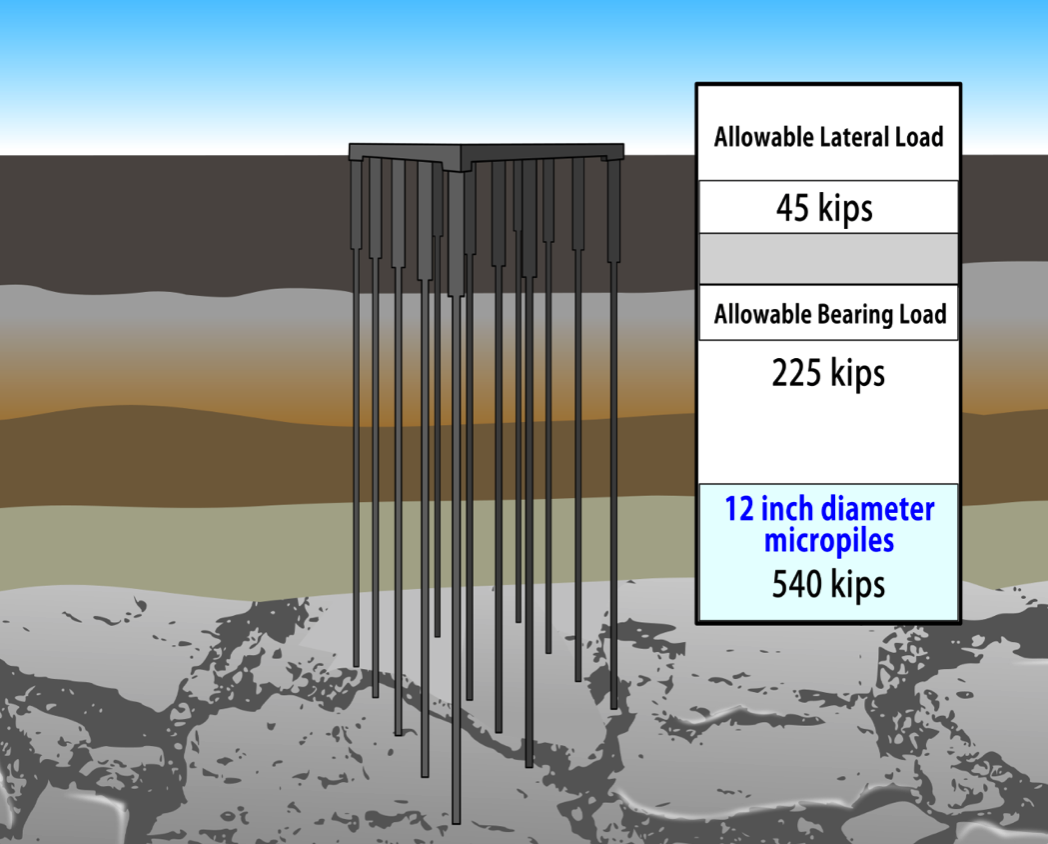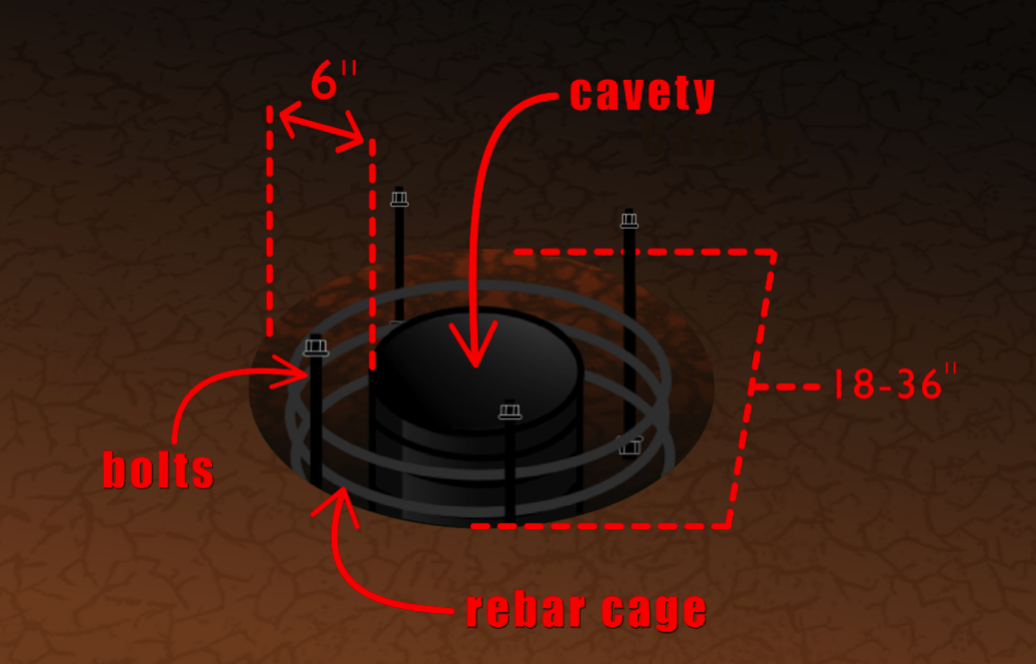Single Source for Integrated Engineering and Construction Services

•••••••# KVC Pile Geotechnical Parameters

KVC Pile – Consolidated Micropile Deep Foundation System invented by Adept Construction Solutions Inc. should be considered for numerous reasons:

• When the upper soil strata are too weak or compressible to support the required vertical loads;

• When shallow foundations cannot adequately support inclined, lateral, or uplift loads, and
overturning moments;

• When soils around foundations are subjected to liquefaction during seismic events (h);

• When there is limited access for large excavation and drilling machinery;

• When future excavations are planned which would require underpinning of shallow foundations;• When expansive or collapsible soils are present, which could cause undesirable seasonal movements of the foundations;

• When conventional driven friction piles are not sufficient to support vertical or lateral loads;

• When large loads are anticipated (compressive, uplift or lateral) and where the amount of
allowable deformation is small;

• When seismically induced downdrag loads are large;

• Where the instability of slope cannot be maintained using conventional methods;

• When there is a limitation on water crossing work;

• When high cost of conventional deep foundation is a factor;• When minimal disturbance is caused during construction.

Sample Evaluation          Geotechnical Capacity of KVC Micropile

The allowable geotechnical bond capacity (PG-allowable)

PG allowable = (αbond/F.S.) x (π x DB x LB)

Where:

αbond = grout to ground ultimate bond strength;

F.S. = factor of safety applied to the ultimate bind strength;

DB = diameter of the bore hole;

LB = bond length12- Inch Diameter

Zone 1:

LB = 20 psi

Length = 35 ft minimum

PG allowable for Zone 1 = (20psi/2) x π x 12 inches x 35 feet x 12 inches = 158 Kips

Zone 2:

αB = 35 psi

Length = 30 ft minimum

Total Minimum Drill Depth = 65 ft

PG allowable for Zone 2 = (35psi/2) x π x 12 inches x 30 feet x 12 inches = 237 Kips

Total allowable 12” KVC micropile (Zone 1+Zone 2) = 396 Kips

9- Inch Diameter

Zone 1:

αB = 20 psi

Length = 35 ft minimum

PG allowable for Zone 1 = (20psi/2) x π x DB x LB

= (20psi/2) x π x 9 inch x 35 feet x 9 inches = 119 Kips

Zone 2:

αB = 35 psi

Length = 30 ft minimum

PG allowable for Zone 2 = (35psi/2) x π x DB x LB

= (35psi/2) x π x 9 inch x 30 feet x 9 inches = 178 Kips

Total Minimum Drill Depth = 65 ft

Total allowable 9” KVC micropile (Zone 1+Zone 2) = 296 Kips

Lateral Load-Bearing Pier 24 Inch Diameter

PG allowable: Vertical Static

Minimum Drill Depth = 16 feet

2 feet x π x 400 psf x 15 feet = 37 Kips

PG allowable: Lateral Static

30 Kips